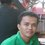# Definitions

Hello Brilliant community! I have here some questions about definitions of mathematical concepts. While most of these do not involve problem-solving or proving (as indicated by the title), you might find some of these challenging - or trivial, depending on your perspective.

1. Why do we have to rationalize the denominator of a fraction if it contains a radical?
2. Does the definition of a kite require it to be a convex polygon?
3. Are all parallelograms trapezoids?
4. Why is $\frac {0} {0}$ indeterminate, but $\frac {1} {0}$ undefined? What is the difference between the two terms? (Wanna go the extra mile? Answer this question without using limits or other calculus concepts.)
5. Trapezoid and trapezium - one has a pair of parallel sides, the other has none. Which is which?Note by Francis Gerard Magtibay
6 years, 1 month ago

This discussion board is a place to discuss our Daily Challenges and the math and science related to those challenges. Explanations are more than just a solution — they should explain the steps and thinking strategies that you used to obtain the solution. Comments should further the discussion of math and science.

When posting on Brilliant:

• Use the emojis to react to an explanation, whether you're congratulating a job well done , or just really confused .
• Ask specific questions about the challenge or the steps in somebody's explanation. Well-posed questions can add a lot to the discussion, but posting "I don't understand!" doesn't help anyone.
• Try to contribute something new to the discussion, whether it is an extension, generalization or other idea related to the challenge.

MarkdownAppears as
*italics* or _italics_ italics
**bold** or __bold__ bold
- bulleted- list
• bulleted
• list
1. numbered2. list
1. numbered
2. list
Note: you must add a full line of space before and after lists for them to show up correctly
paragraph 1paragraph 2

paragraph 1

paragraph 2

[example link](https://brilliant.org)example link
> This is a quote
This is a quote
    # I indented these lines
# 4 spaces, and now they show
# up as a code block.

print "hello world"
# I indented these lines
# 4 spaces, and now they show
# up as a code block.

print "hello world"
MathAppears as
Remember to wrap math in $$ ... $$ or $ ... $ to ensure proper formatting.
2 \times 3 $2 \times 3$
2^{34} $2^{34}$
a_{i-1} $a_{i-1}$
\frac{2}{3} $\frac{2}{3}$
\sqrt{2} $\sqrt{2}$
\sum_{i=1}^3 $\sum_{i=1}^3$
\sin \theta $\sin \theta$
\boxed{123} $\boxed{123}$

Sort by:

4) $\frac { 0 }{ 0 }$ may have multiple finite values, while $\frac { 1 }{ 0 }$ cannot be expressed by any finite value, as $0$ times any finite value is $0$, a contradiction.

- 6 years, 1 month ago

5) "Trapezium" is the messed up one, there isn't an universal agreement on what it means. It can mean a quadrilateral with two parallel sides or with no pair of parallel sides. Everybody agrees that "Trapezoid" means a quadrilateral with two parallel sides.

- 6 years, 1 month ago

I thought this was a matter of differences between American and British English, because Wolfram said so. Thanks anyway, Mr. Mendrin. :)

- 6 years, 1 month ago

We all know that the British drive on the wrong side of the road too.

- 6 years, 1 month ago

This just made my day. :) No offense, British-English speakers. :)

- 6 years, 1 month ago

1) Who says we have to rationalize the denominator of a fraction if it contains a radical (or anything else for that matter)? The only justification for it would be that it could lead to wrong results of one doesn't, but it won't. It just makes further manipulation or numerical computation easier if we do--but not necessarily always easier. It depends.

- 6 years, 1 month ago

So far I've found three possible answers to this question.

1. It's simply a matter of preference!

2. It's much easier to add two fractions with different denominators if these happen to be integers rather than radicals. For instance, $\frac {\sqrt{2}}{2} + \frac {\sqrt{3}}{3}$ is much easier to evaluate than its equivalent $\frac {1}{\sqrt{2}} + \frac {1}{\sqrt{3}}$.

3. Rational numbers are defined as numbers of the form $\frac {p}{q}$ where $p, q \in \mathbb {Z}$ and $q \neq 0$. It follows that the denominator of a rational number is always a nonzero integer, If we multiply a rational number $\frac {p}{q}$ by a radical $r$, the product $\frac {pr}{q}$ will always contain a radical in the numerator, not the denominator.

The first two are a bit related, and the third might have some logical loopholes. Feel free to comment!

- 6 years, 1 month ago

There's a distinction between "have to" and "ought to". Yes, we ought to rationalize the denominator, but at the end, it doesn't lead to wrong results if we don't bother to.

- 6 years, 1 month ago

Indeed.

- 6 years, 1 month ago

3) Yes

- 6 years, 1 month ago

But different sources provide different definitions of the term trapezoid. Some say it has exactly one pair of parallel sides, while according to others, it has at least one such pair of sides. So does it just depend on which definition we use? Or is there something wrong with one of them?

- 6 years, 1 month ago

2) I leave this one to the reader as an exercise.

Edit: Okay, since nobody's answering this one, a kite is a quadrilateral that has two pairs of sides of equal length, but with each such pair of equal length sides sharing a common vertex. Contrast this with a parallelogram, where no such pair of equal length sides share a common vertex. This doesn't preclude a kite that is non-convex, i.e., it can vaguely look like a Chevron or maybe an paleolithic arrowhead.

- 6 years, 1 month ago